## 3.1 Krawczyk の定理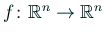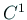級として、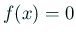を満たすを求める。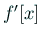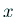における微分、すなわちヤコビ行列とする: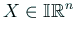に対して、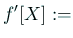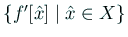の区間包囲

とおく。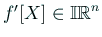である。

 要約 Newton-Algorithms for Evaluation of Roots with Error Bounds. In this paper some definitions and properties of interval-norms and spans are treated. Then several NEw oN-Mgorithms for finding roots of functions with one variable are given. The algorithms use interval-arithmetics and yield an interval-result containing the exac  root. The algorithms then are generalized to solve systems of equations.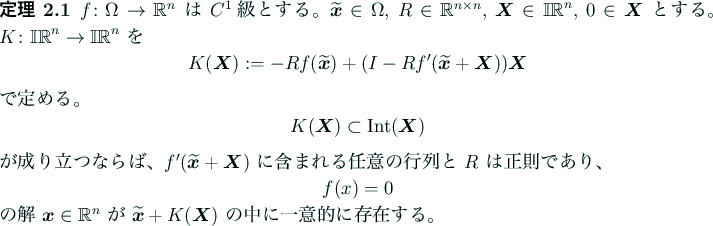2020-09-03